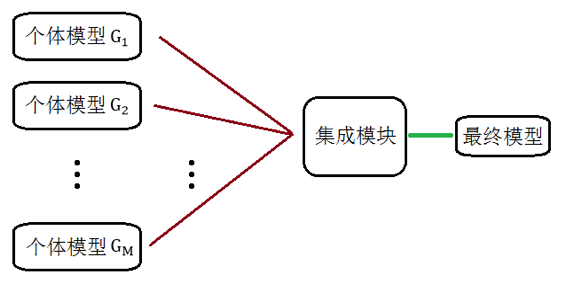# 众擎易举• 将不同类型的弱分类器进行提升
• 将相同类型但参数不同的弱分类器进行提升
• 将相同类型但训练集不同的弱分类器进行提升

# Bagging 与随机森林

Bagging 是 1996 年由 Breiman 提出的，它的思想根源是数理统计中非常重要的 Bootstrap 理论。Bootstrap 可以翻译成“自举”，它通过模拟的方法来逼近样本的概率分布函数。可以想象这样一个场景：现在有一个包含 N 个样本的数据集$X = \{ x_{1},\ldots,x_{N}\}$，这 N 个样本是由随机变量$x$独立生成的。我们想要研究$x$的均值估计$\bar{x} = \frac{1}{N}\sum_{i = 1}^{N}x_{i}$的统计特性（误差、方差等等），但由于研究统计特性是需要大量样本的、而数据集$X$只能给我们提供一个$\bar{x}$的样本，从而导致无法进行研究

• $X$中随机抽出一个样本（亦即抽出$x_1,...,x_N$的概率相同）
• 将该样本的拷贝放入数据集$X_j$
• 将该样本放回$X$

• Bootstrap 以$\frac 1N$的概率、有放回地从$X$中抽取 N 个样本作为数据集、并以之估计真实分布生成的具有 N 个样本的数据集
• 经验分布函数则是在 N 个样本点上以每点的概率为$\frac 1N$作为概率密度函数、然后进行积分的函数

• 用 Bootstrap 生成出 M 个数据集
• 用这 M 个数据集训练出 M 个弱分类器
• 最终模型即为这M个弱分类器的简单组合

• 对于分类问题使用简单的投票表决
• 对于回归问题则进行简单的取平均

• 用 Bootstrap 生成出 M 个数据集
• 用这 M 个数据集训练出 M 颗不进行后剪枝决策树，且在每颗决策树的生成过程中，每次对 Node 进行划分时、都从可选特征（比如说有 d 个）中随机挑选出 k 个（$k\le d$）特征，然后依信息增益的定义从这 k 个特征中选出信息增益最大的特征作为划分标准
• 最终模型即为这 M 个弱分类器的简单组合

• $k=d$，那么训练出来的决策树和一般意义下的决策树别无二致、亦即特征选取这一部分不具有随机性
• $k=1$，那么生成决策树的每一步都是在随机选择属性、亦即特征选取的随机性达到最大

Breiman 在提出随机森林算法的同时指出，一般情况下、推荐取$k=\log_2{d}$

# PAC 框架与 Boosting

PAC 学习整体来说是一个比较纯粹的数学理论。有一种说法是、PAC 学习是统计学家研究机器学习的方式，它关心模型的可解释性、然而机器学习专家通常更关心模型的预测能力。这也正是为何说无需太过了解它，因为我们的目的终究不是成为统计的专家、而是更希望成为一个能够应用机器学习的人。不过幸运的是，虽然为了叙述 Boosting、PAC 学习中“可学习性”的概念难以避开，但其本身却是具有很直观的解释的。下面我们就来看看这个直观解释：

PAC 提出的一个主要的假设、就是它要求数据是从某个稳定的概率分布中产生。直观地说，就是样本在样本空间中的分布状况不能随时间的变化而变化、否则就失去了学习的意义（因为学习到的永远只是“某个时间”的分布，如果未知数据所处时间的分布状况和该时间数据的分布状况不同的话、模型就直接失效了）。然后所谓的PAC可学习性，就是看学习的算法是否能够在合理的时间（多项式时间）内、以足够高的概率输出一个错误率足够低的模型。由此，所谓的“强可学习”和“弱可学习”的概念就很直观了：

• 若存在一个多项式算法可以学习出准确率很高的模型，则称为强可学习
• 若在在一个多项式算法可以学习但准确率仅仅略高于随机猜测，则称为弱可学习

• 根据当前的数据训练出一个弱模型
• 根据该弱模型的表现调整数据的样本权重。具体而言：
• 让该弱模型做错的样本在后续训练中获得更多的关注
• 让该弱模型做对的样本在后续训练中获得较少的关注
• 最后再根据该弱模型的表现决定该弱模型的“话语权”、亦即投票表决时的“可信度”。自然、表现越好就越有话语权